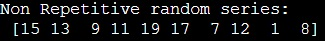# Non-repetitive random number in NumPy

Learn, how to generate a non-repetitive random series in numpy?
Submitted by Pranit Sharma, on January 05, 2023

NumPy is an abbreviated form of Numerical Python. It is used for different types of scientific operations in python. Numpy is a vast library in python which is used for almost every kind of scientific or mathematical operation. It is itself an array which is a collection of various methods and functions for processing the arrays.

## NumPy - Generating a non-repetitive random series

The numpy.random() method is used to generate some random numbers which may get repeated but here, we need to generate a non-repetitive random number series.

In numpy random module, we have a method default_rng() which will return an object, this object contains a method called "choice() with the help of which we can create a random number series and if we pass an argument called replace=False, it will create a non repetitive random number series.

Let us understand with the help of an example,

## Python code to generate a non-repetitive random series in numpy

```# Import numpy
import numpy as np

# Import default_rng
from numpy.random import default_rng

# Creating an object of default_rng
obj = default_rng()

# Creating non repetitive random series
res = obj.choice(20, size=10, replace=False)

# Display result
print("Non Repetitive random series:\n",res)
```

Output: